JalFaizy Shaikh — Published On October 5, 2017 and Last Modified On October 16th, 2020

## Introduction

Extracting useful information from unstructured data has always been a topic of huge interest in the research community. One such example of unstructured data is an image, and analysis of image data has applications in various aspects of business.

This skilltest is specially designed for you to test your knowledge on the knowledge on how to handle image data, with an emphasis on image processing. More than 300 people registered for the test. If you are one of those who missed out on this skill test, here are the questions and solutions.

Here is the leaderboard for the participants who took the test.

Here are some resources to get in depth knowledge in the subject.

## Skill test Questions and Answers

1) Match the following image formats to their correct number of channels

• GrayScale
• RGB
1.  1 channel
2. 2 channels
3. 3 channels
4. 4 channels

None
A) RGB -> I, GrayScale-> III
B) RGB -> IV, GrayScale-> II
C) RGB -> III, GrayScale -> I
D) RGB -> II, GrayScale -> I

2)

Suppose you have to rotate an image. Image rotation is nothing but multiplication of image by a specific matrix to get a new transformed image.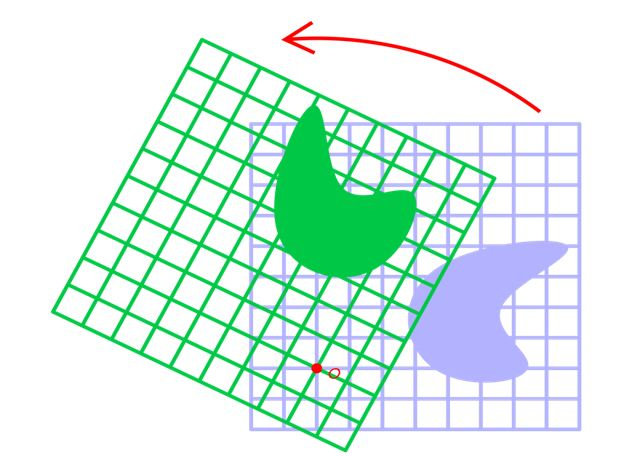For simplicity, we consider one point in the image to rotate with co-ordinates as (1, 0) to a co-ordinate of (0, 1), which of the following matrix would we have to multiply with?

3) [True or False] To blur an image, you can use a linear filter

A) TRUE
B) FALSE

4) Which of the following is a challenge when dealing with computer vision problems?

A) Variations due to geometric changes (like pose, scale etc)
B) Variations due to photometric factors (like illumination, appearance etc)
C) Image occlusion
D) All of the above

5)

Suppose we have an image given below.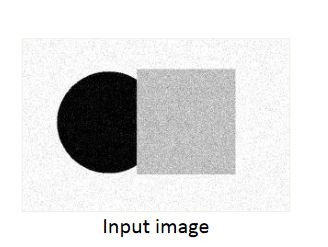Our task is to segment the objects in the image. A simple way to do this is to represent the image in terms of the intensity of pixels and the cluster them according to the values. On doing this, we got this type of structure.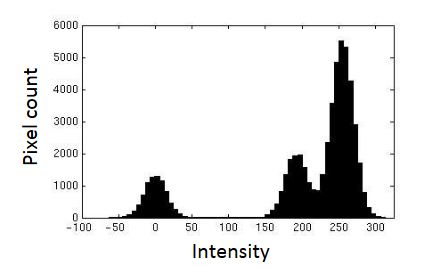Suppose we choose k-means clustering to solve the problem, what would be the appropriate value of k from just a visual inspection of the intensity graph?

A) 1
B) 2
C) 3
D) 4

6)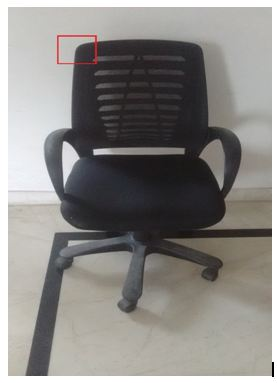In this image, you can find an edge labelled in the red region. Which form of discontinuity creates this kind of edge?

A) Depth Discontinuity
B) Surface color Discontinuity
C) Illumination discontinuity
D) None of the above

Solution: A

The chair and wall are far from each other, causing an edge in the image.

7) Finite difference filters in image processing are very susceptible to noise. To cope up with this, which of the following methods can you use so that there would be minimal distortions by noise?

A) Downsample the image
B) Convert the image to grayscale from RGB
C) Smooth the image
D) None of the above

Solution: C

Smoothing helps in reducing noise by forcing pixels to be more like their neighbours

8) Consider and image with width and height as 100×100. Each pixel in the image can have a color from Grayscale, i.e. values. How much space would this image require for storing?

Note: No compression is done.

A) 2,56,00,000
B) 25,60,000
C) 2,56,000
D) 8,00,000
E) 80,000
F) 8,000

Solution: E

The answer will be 8x100x100 because 8 bits will be required to represent a number from 0-256

9) [True or False] Quantizing an image will reduce the amount of memory required for storage.

A) TRUE
B) FALSE

Solution: A

The statement given is true.

10) Suppose we have a grayscale image, with most of the values of pixels being same. What can we use to compress the size of image?

A) Encode the pixels with same values in a dictionary
B) Encode the sequence of values of pixels
C) No compression can be done

Solution: A

Encoding same values of pixels will greatly reduce the size for storage

11) [True or False] JPEG is a lossy image compression technique

A) TRUE
B) FALSE

Solution: A

The reason for JPEG being a lossy compression technique is because of the use of quantization.

12) Given an image with only 2 pixels and 3 possible values for each pixel, what is the number of possible image histograms that can be formed?

A) 3
B) 6
C) 9
D) 12

Solution: C

The permutations possible of the histograms would be 9.

13) Suppose we have a 1D image with values as

[2, 5, 8, 5, 2]

Now we apply average filter on this image of size 3. What would be the value of the last second pixel?

A) The value would remain the same
B) The value would increase by 2
C) The value would decrease by 2
D) None of the above

Solution: A

(8+5+2)/3 will become 5. So there will be no change.

14) fMRI (Functional magnetic resonance imaging) is a technology where volumetric scans of the brain are acquired while the subject is performing some cognitive tasks over time. What is the dimensionality of fMRI output signals?

A) 1D
B) 2D
C) 3D
D) None of the above

Solution: D

The question itself mentions “volumetric scans” over time, so it would be a series of 3D scans

15) Which of the following methods is used as a model fitting method for edge detection?

A) SIFT
B) Difference of Gaussian detector
C) RANSAC
D) None of the above

Solution: C

RANSAC is used to find the best fit line in edge detection

16)

Suppose we have an image which is noisy. This type of noise in the image is called salt-and-pepper noise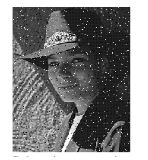[True or False] Median filter technique is the best way to denoise this image

A) TRUE
B) FALSE

Solution: A

Median filter technique helps reduce noise to a good enough extent

17) If we convolve an image with the matrix given below, what would be the relation between the original and modified image?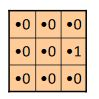A) The image will be shifted to the right by 1 pixel
B) The image will be shifted down by 1 pixel
C) The image will be shifted to the left by 1 pixel
D) The image will be shifted up by 1 pixel

Solution: A

I would suggest you to try this yourself and see the result!

18) Which of the following is a correct way to sharpen an image?

A)

1. Convolve the image with identity matrix
2. Subtract this resulting image from the original
3. Add this subtracted result back to the original image

B)

1. Smooth the image
2. Subtract this smoothed image from the original
3. Add this subtracted result back to the original image

C)

1. Smooth the image
2. Add this smoothed image back to the original image

D) None of the above

Solution: B

Option B gives a correct way to sharpen an image

19) Below given images are two operations performed on a signal. Can you identify which is which?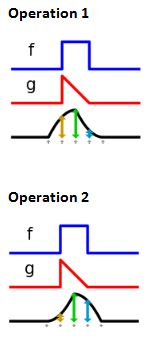A) Operation 1 is cross correlation between signal f and signal g, whereas operation 2 is convolution function applied to signal f and signal g
B) Operation 2 is cross correlation between signal f and signal g, whereas operation 1 is convolution function applied to signal f and signal g

Solution: A

Correlation and convolution are two different methods with give different result. Convolution defines how much the signals overlap, whereas correlation tries to find the relation between the signals

20) [True or False] By using template matching along with cross correlation, you can build a vision system for TV remote control

A) TRUE
B) FALSE

Solution: A

This is a excellent example of cross correlation in computer vision. Refer paper “Computer Vision for Interactive Computer Graphics,” W.Freeman et al, IEEE Computer Graphics and Applications

21) Suppose you are creating a face detector in the wild. Which of the following features would you select for creating a robust facial detector?

1. Location of iris, eyebrow and chin
2. Boolean feature: Is the person smiling or not
3. Angle of orientation of face
4. Is the person sitting or standing

A) 1, 2
B) 1, 3
C) 1, 2, 3
D) 1, 2, 3, 4

Solution: B

Options 1, 3 would be relevant features for the problem, but 2, 4 may not be

22) Which of the following is example of low level feature in an image?

A) HOG
B) SIFT
C) HAAR features
D) All of the above

Solution: D

All the above are examples of low-level features

23) In RGBA mode of color representation, what does A represent?

A) Depth of an image
B) Intensity of colors
C) Opacity of an image
D) None of the above

Solution: C

Opacity can be mentioned by introducing it as a fourth parameter in RGB

24) In Otsu thresholding technique, you remove the noise by thresholding the points which are irrelevant and keeping those which do not represent noise.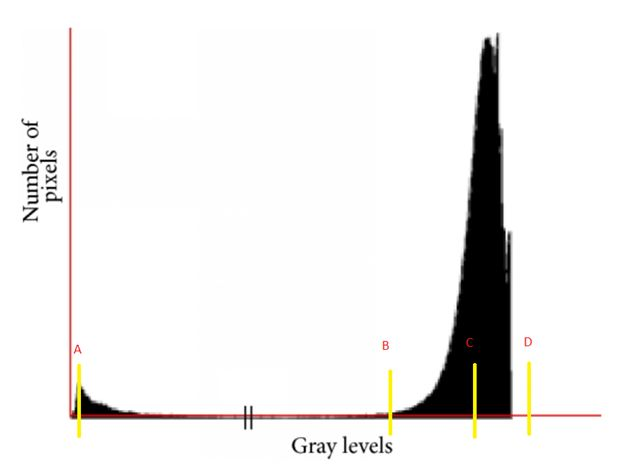In the image given, at which point would you threshold on?

A) A
B) B
C) C
D) D

Solution: B

Line B would catch most of the noise in the image.

25) Which of the following data augmentation technique would you prefer for an object recognition problem?

`
A) Horizontal flipping
B) Rescaling
C) Zooming in the image
D) All of the above

Solution: D

All the mentioned techniques can be used for data augmentation.

## Overall Distribution

Below is the distribution of the scores of the participants: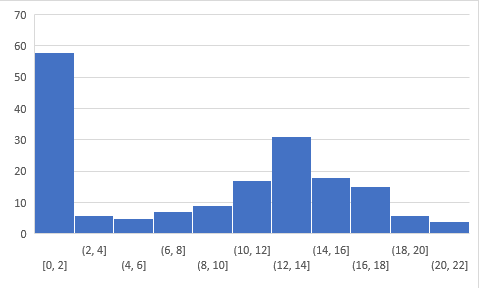You can access the scores here. More than a hundered people participated in the skill test and the highest score obtained was a 22.

## End Notes

I tried my best to make the solutions as comprehensive as possible but if you have any questions / doubts please drop in your comments below. I would love to hear your feedback about the skill test.

### Learn, engage,compete, and get hired!###### JalFaizy Shaikh

Faizan is a Data Science enthusiast and a Deep learning rookie. A recent Comp. Sc. undergrad, he aims to utilize his skills to push the boundaries of AI research.